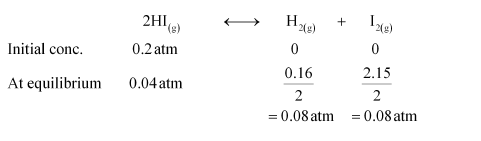# A sample of HI(g) is placed in flask at a pressure of 0.2 atm.

Question:

A sample of $\mathrm{HI}_{(\mathrm{g})}$ is placed in flask at a pressure of $0.2$ atm. At equilibrium the partial pressure of $\mathrm{HI}_{(\mathrm{g})}$ is $0.04$ atm. What is $K_{\mathrm{p}}$ for the given equilibrium?

$2 \mathrm{HI}(\mathrm{g}) \longleftrightarrow \mathrm{H}_{2}(\mathrm{~g})+\mathrm{I}_{2}(\mathrm{~g})$

Solution:

The initial concentration of $\mathrm{HI}$ is $0.2 \mathrm{~atm}$. At equilibrium, it has a partial pressure of $0.04 \mathrm{~atm}$. Therefore, a decrease in the pressure of $\mathrm{HI}$ is $0.2-0.04=0.16$. The given reaction is:Therefore,

$K_{\mathrm{p}}=\frac{p_{\mathrm{H}_{2}} \times p_{\mathrm{I}_{2}}}{p_{\mathrm{HI}}^{2}}$

$=\frac{0.08 \times 0.08}{(0.04)^{2}}$

$=\frac{0.0064}{0.0016}$

$=4.0$

Hence, the value of Kp for the given equilibrium is 4.0.